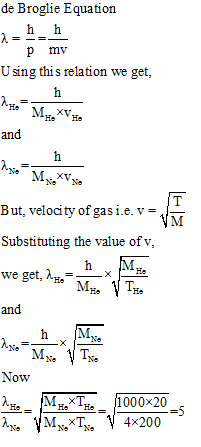Courses

# Notes | EduRev

## JEE : Notes | EduRev

The document Notes | EduRev is a part of the JEE Course NEET Revision Notes.
All you need of JEE at this link: JEE

Question 1: Energy of an electron is given by E = -2.178×10-18 J Z2/n2. Wavelength of light required to excite an electron in an hydrogen atom from level n =1 to n = 2 will be (h = 6.62 ×10−34 Js and c = 3.0 ×10ms−1)   (IIT JEE-2013)
(a) 2.816 ×10−7 m
(b) 6.500 ×10−7m
(c) 8.500 ×10−7m
(d) 1.214 ×10−7m
Answer: d
Solution:
We know that energy of an electron can be calculated using Planck relation
E = hc/λ     …………… (i)
But in the question it is given that
E = -2.178 × 10-18 J Z2 (1/n12 -1/n22) …………(ii)
On comparing both the equations we get,
hc /λ =-2.178×10-18 J Z2(1/n12 - 1/n22) ………….(iii)
On substituting the values
h =6.62 ×10−34 Js
c = 3.0 ×10ms−1
n1= 1 & n= 2
In equation (iii) and calculating for the value of λ we get,
λ =1.214 ×10−7m
Hence, the correct option is d.

Question 2: The atomic masses of He and Ne are 4 and 20 a.m.u., respectively. The value of the de Broglie wavelength of He gas at –73 oC is “M” times that of the de Broglie wavelength of Ne at 727oC. M is  (IIT JEE-2013)
Solution:Question 3: The kinetic energy of an electron in the second Bohr orbit of a hydrogen atom is [a0 is Bohr radius] (IIT JEE 2012)
(a) h2/4π2m a02
(b) h2/16π2m a02
(c) h2/32π2m a02
(d) h2/64π2m a02
Answer: c
Solution:
According to Bohr’s postulate,
Electrons can exist only in those orbital around the nucleus, for which the angular momentum is integral multiple of  h/2π i.e.
mvr  = n h/2π
or,
v = n h/2πmr
So, Kinetic Energy of electrons = ½ m (n h/2πmr)2
Radius of the orbital i.e. rn = (a0×n2)/Z
For, n=2 & z =1
r = 4 a0
So,
Kinetic Energy of electrons = ½ m [4 h2/(4π2m2(4 a0))]2 = h2/32π2m a02
Hence, the correct option is c.

Offer running on EduRev: Apply code STAYHOME200 to get INR 200 off on our premium plan EduRev Infinity!

291 docs

,

,

,

,

,

,

,

,

,

,

,

,

,

,

,

,

,

,

,

,

,

;